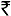Mathematical QB550.00520.00
Expiry : 180 Days
Exams : Applications of Differential Equations QB-1 | Complex Analysis QB-1 | Complex Analysis QB-2 | Differential Equations QB-1 | Differential Equations Revision QB-1 | Differential Equations, Legendre Polynomials QB-1 | Fourier Series, Laplace Transform, Numerical Analysis QB-1 | Laplacian, Radial Forms, Line, Surface, Volume Integral QB-1 | Linear Algebra QB-1 | Linear Algebra QB-2 | Second order Differential Equations QB-2 | Vector Calculus and Linear Algebra QB-1 | Vector Calculus and Linear Algebra QB-2 | Complete Maths QB-2 |

## Product Description

This Package is only for UV DEC CSIR Batch Students ( Not for Others, Please Don't Purchase)